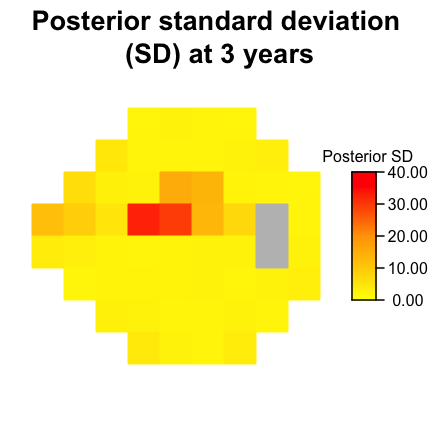# Introduction to using R package: spBFA

## Use of spBFA

This is a brief description of how to use the spBFA package within the context of glaucoma progression. To demonstrate the method we use the VFSeries data set from the womblR package. We begin by loading the packages.

library(womblR)
library(spBFA)
##
## Attaching package: 'spBFA'
## The following object is masked from 'package:womblR':
##
##     diagnostics

The data object VFSeries has four variables, Visit, DLS, Time and Location. The data object loads automatically; here’s what the data looks like,

head(VFSeries)
##   Visit DLS Time Location
## 1     1  25    0        1
## 2     2  23  126        1
## 3     3  23  238        1
## 4     4  23  406        1
## 5     5  24  504        1
## 6     6  21  588        1

The variable Visit represents the visual field test visit number, DLS the observed outcome variable, differential light sensitivity, Time the time of the visual field test (in days from baseline visit) and Location the spatial location on the visual field that the observation occurred. To help illuminate visual field data we can use the PlotVFTimeSeries function from the womblR package. PlotVFTimeSeries is a function that plots the observed visual field data over time at each location on the visual field.

PlotVfTimeSeries(Y = VFSeries$DLS, Location = VFSeries$Location,
Time = VFSeries$Time, main = "Visual field sensitivity time series \n at each location", xlab = "Days from baseline visit", ylab = "Differential light sensitivity (dB)", line.col = 1, line.type = 1, line.reg = FALSE)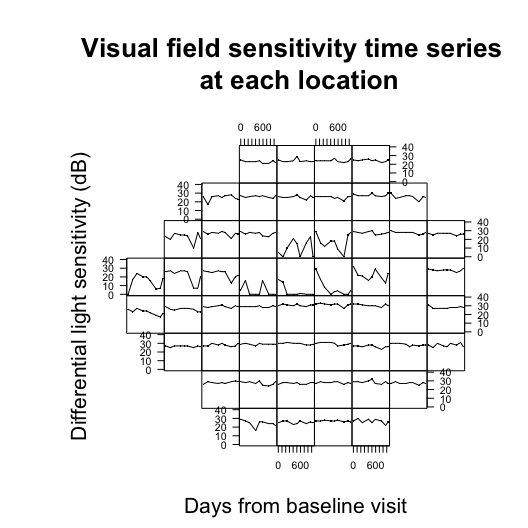The figure above demonstrates the visual field from a Humphrey Field Analyzer-II testing machine, which generates 54 spatial locations (only 52 informative locations, note the 2 blanks spots corresponding to the blind spot). At each visual field test a patient is assessed for vision loss. ## Format data for spBFA We can now begin to think about preparing objects for use in the Bayesian spatial factor analysis function (bfa_sp). According to the manual, we use a formula specification for the observed data, where the data must be first ordered spatially and then temporally (We only use on spatial observation type in this example, thus O is one.). Furthermore, we will remove all locations that correspond to the natural blind spot (which in the Humphrey Field Analyzer-II correspond to locations 26 and 35). blind_spot <- c(26, 35) # define blind spot VFSeries <- VFSeries[order(VFSeries$Location), ] # sort by location
VFSeries <- VFSeries[order(VFSeries$Visit), ] # sort by visit VFSeries <- VFSeries[!VFSeries$Location %in% blind_spot, ] # remove blind spot locations
dat <- data.frame(Y = VFSeries$DLS / 10) # create data frame with scaled data Now that we have assigned the observed outcome we move onto the temporal variable time. For visual field data we define this to be the time from the baseline visit. We obtain the unique days from the baseline visit and scale them to be on the year scale. Time <- unique(VFSeries$Time) / 365 # years since baseline visit
print(Time)
##  0.0000000 0.3452055 0.6520548 1.1123288 1.3808219 1.6109589 2.0712329
##  2.3780822 2.5698630

Our example patient has nine visual field visits and the last visit occurred 2.57 years after the baseline visit.

We now specify the adjacency matrix, W, for our areal data analysis. We use the HFAII_Queen adjacency matrix for visual fields, provided in womblR. This is an adjacency matrix that defines an adjacency as edges and corners (i.e., the movements of a queen in chess). The adjacency objects are preloaded and contain the blind spot, so we define our adjacency matrix as follows.

W <- HFAII_Queen[-blind_spot, -blind_spot] # visual field adjacency matrix
M <- dim(W) # number of locations

Now that we have specified the data objects, we will customize the objects that characterize Bayesian Markov chain Monte Carlo (MCMC) methods, in particular hyperparameters, starting values, metropolis tuning values and MCMC inputs.

## MCMC Characteristics

We begin be specifying the hyperparameters for the model. The parameter $$\psi$$ is uniformly distributed with lower bound, $$a_{\psi}$$, and upper bound, $$b_{\psi}$$. The upper bound for $$\psi$$ cannot be specified arbitrarily since it is important to account for the magnitude of time elapsed. We specify the following upper bound for $$\psi$$ to dictate a weakly informative prior distribution.

TimeDist <- as.matrix(dist(Time))
BPsi <- log(0.025) / -min(TimeDist[TimeDist > 0])
APsi <- log(0.975) / -max(TimeDist)

Then, we can create a hyperparameters list object, Hypers, that can be used for spBFA. We first define the number of latent factors (K) and spatial observation types (O).

K <- 10
O <- 1
Hypers <- list(Sigma2 = list(A = 0.001, B = 0.001),
Kappa = list(SmallUpsilon = O + 1, BigTheta = diag(O)),
Delta = list(A1 = 1, A2 = 20),
Psi = list(APsi = APsi, BPsi = BPsi),
Upsilon = list(Zeta = K + 1, Omega = diag(K)))

Here, $$\sigma2$$ has an inverse Gamma distribution with shape and scale, $$\kappa$$ and $$\Upsilon$$ have inverse-Wishart distributions with degrees of freedom (scalar) and scale matrix, and $$\delta$$ has a multiplicative gamma process shrinkage prior (See the help manual for spBFA for further details).

Specify a list object, Starting, that contains the starting values for the hyperparameters.

Starting <- list(Sigma2 = 1,
Kappa = diag(O),
Delta = 2 * (1:K),
Psi = (APsi + BPsi) / 2,
Upsilon = diag(K))

Provide tuning parameters for the metropolis steps in the MCMC sampler.

Tuning <- list(Psi = 1)

We set Tuning to the default setting of one and let the pilot adaptation in the burn-in phase tune the acceptance rates to the appropriate range. Finally, we set the MCMC inputs using the MCMC list object.

MCMC <- list(NBurn = 1000, NSims = 1000, NThin = 2, NPilot = 5)

We specify that our model will run for a burn-in period of 1,000 scans, followed by 1,000 scans after burn-in. In the burn-in period there will be 5 iterations of pilot adaptation evenly spaced out over the period. Finally, the final number of samples to be used for inference will be thinned down to 500 based on the thinning number of 2. We suggest running the sampler 250,000 iterations after burn-in, but in the vignette we are limited by compilation time.

## Bayesian spatial factor analysis

We have now specified all model objects and are prepared to implement the bfa_sp regression object. To demonstrate the bfa_sp object we will use all of its options, even those that are being used in their default settings.

reg.bfa_sp <- bfa_sp(Y ~ 0, data = dat, dist = W, time = Time,  K = 10,
starting = Starting, hypers = Hypers, tuning = Tuning, mcmc = MCMC,
L = Inf,
family = "tobit",
trials = NULL,
temporal.structure = "exponential",
spatial.structure = "discrete",
seed = 54,
gamma.shrinkage = TRUE,
include.space = TRUE,
clustering = TRUE)

## Burn-in progress:  |*************************************************|
## Sampler progress:  0%..  10%..  20%..  30%..  40%..  50%..  60%..  70%..  80%..  90%..  100%..

The first line of arguments are the data objects, formula, data, dist, time, and K. These objects must be specified for bfa_sp to run. Note that in the formula we use ~0, which means that there are no covariates. The second line of objects are the MCMC characteristics objects we defined previously. These objects do not need to be defined for spBFA to function, but are provided for the user to customize the model to their choosing. If they are not provided, defaults are given. Next, we specify that the infinite mixture model is used L = Inf. L can be any positive integer or infinity. The family is set to tobit since we know that visual field data is censored (family can be normal, probit, tobit, or binomial). If binomial is used, trials must be specified. Our temporal dependence structure is set to be exponential, and the spatial structure is discrete (i.e., areal). Finally, we define the following logicals, gamma.shrinkage (which controls the gamma shrinkage process), include.space (which controls if spatial dependency is included), and clustering (which controls if the Bayesian non-parametric process prior is used). We set the seed to 54.

The following are the returned objects from reg.bfa_sp.

names(reg.bfa_sp)
##   "lambda"     "eta"        "beta"       "sigma2"     "kappa"
##   "delta"      "tau"        "upsilon"    "psi"        "xi"
##  "rho"        "metropolis" "datobj"     "dataug"     "runtime"

The object reg.bfa_sp contains raw MCMC samples for all parameters, metropolis acceptance rates and final tuning parameters (metropolis) and model runtime (runtime). The objects datobj and dataug can be ignored as they are for later use in secondary functions.

## Assessing model convergence

Before analyzing the raw MCMC samples from our model we want to verify that there are no convergence issues. We begin by loading the coda package.

library(coda)

Then we convert the raw spBFA MCMC objects to coda package mcmc objects. We look at $$\sigma^2(\mathbf{s}_1)$$ only for learning purposes.

Sigma2_1 <- as.mcmc(reg.bfa_sp$sigma2[, 1]) We begin by checking traceplots of the parameter.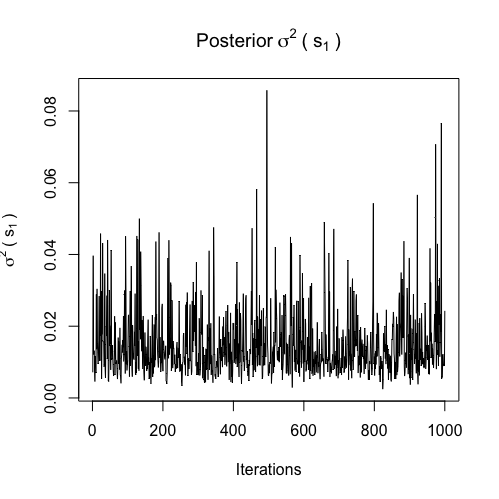From the figure, it is clear that the traceplots exhibit some poor behavior. However, these traceplots are nicely behaved considering the number of iterations the MCMC sampler ran. The traceplots demonstrate that the parameters have converged to their stationary distribution, but still need more samples to rid themselves of autocorrelation. Finally, we present the corresponding test statistics from the Geweke diagnostic test. ## var1 ## 0.6828447 Since the test statistic is not terribly large in the absolute value there is not strong evidence that our model did not converge. ## Compute diagnostics The diagnostics function in the spBFA package can be used to calculate various diagnostic metrics. The function takes in the spBFA regression object. Diags <- spBFA::diagnostics(reg.bfa_sp, diags = c("dic", "dinf", "waic"), keepDeviance = TRUE) ## Calculating Log-Lik: 0%.. 25%.. 50%.. 75%.. 100%.. Done! ## Calculating PPD: 0%.. 25%.. 50%.. 75%.. 100%.. Done! The diagnostics function calculates diagnostics that depend on both the log-likelihood and posterior predictive distribution. So, if any of these diagnostics are specified, one or both of these must be sampled from. The keepDeviance and keepPPD indicate whether or not these distributions should be saved for the user. We indicate that we would like the output to be saved for the log-likelihood (i.e., deviance). We explore the output by looking at the traceplot of the deviance. Deviance <- as.mcmc(Diags$deviance)
traceplot(Deviance, ylab = "Deviance", main = "Posterior Deviance")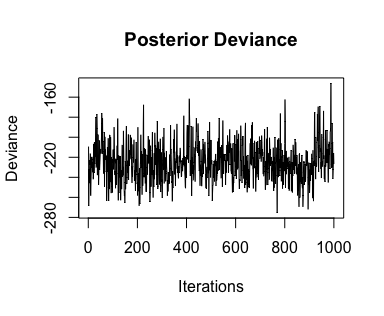This distribution has possible convergence issues, however this is not concerning given the number of MCMC iterations run.

print(Diags)
##       dic        pd
## -100.5787  124.2647
##        p        g     dinf
## 214.9629  66.5324 281.4953
##      waic    p_waic      lppd  p_waic_1
## -92.93843  84.67741 131.14662  37.44991

## Future prediction

The spBFA package provides the predict.spBFA function for sampling from the posterior predictive distribution at future time points of the observed data. This is different from the posterior predictive distribution obtained from the diagnostics function, because that distribution is for the observed time points and is automatically obtained given the posterior samples from spBFA. We begin by specifying the future time point we want to predict as 3 years.

NewTimes <- 3

Then, we use predict.spBFA to calculate the future posterior predictive distribution.

Predictions <- predict(reg.bfa_sp, NewTimes)
## Krigging Eta: 0%.. 25%.. 50%.. 75%.. 100%.. Done!
## Krigging Y: 0%.. 25%.. 50%.. 75%.. 100%.. Done!

We can see that predict.spBFA returns a list containing a matrix of predictions corresponding to each future time point. The name of each matrix is the numeric time point for each future visit.

names(Predictions)
##  "Eta" "Y"

You can plot a heat map representation of the posterior distribution of the change points using the function PlotSensitivity from womblR.

PlotSensitivity(Y = apply(Predictions$Y$Y10, 2, mean) * 10,
main = "Posterior mean prediction\n at 3 years",
legend.lab = "Posterior Mean", legend.round = 2,
bins = 250, border = FALSE, zlim = c(0, 40))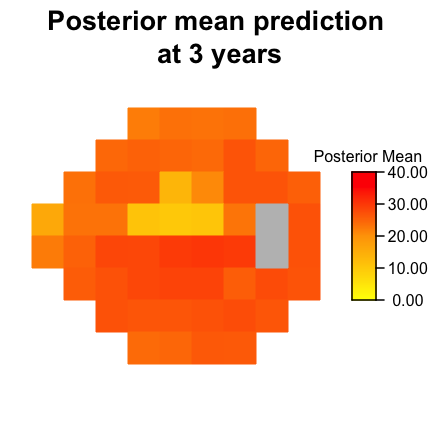This figure shows the posterior predicted mean at 3 years over the visual field. The PlotSensitivity function can be used for plotting any observations on the visual field surface. We can also plot the standard deviation.

PlotSensitivity(Y = apply(Predictions$Y$Y10 * 10, 2, sd),
main = "Posterior standard deviation\n (SD) at 3 years",
legend.lab = "Posterior SD", legend.round = 2,
bins = 250, border = FALSE, zlim = c(0, 40))